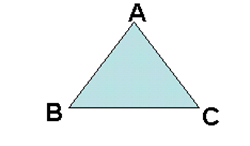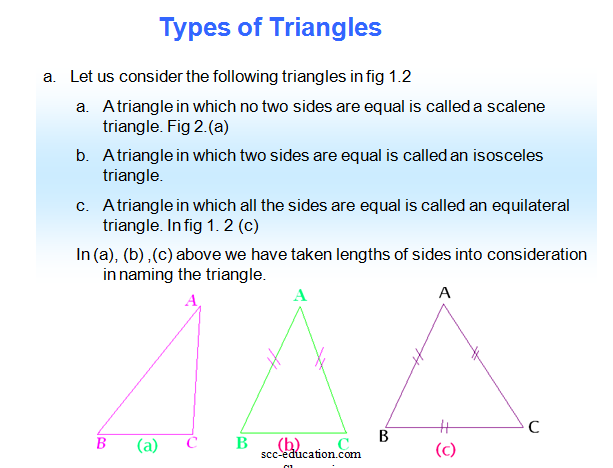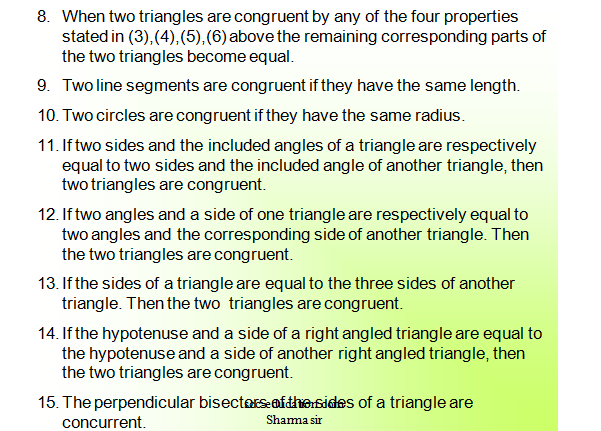# SCC Education

## TRIANGLES and its type and properties

TRIANGLES
Triangle: Triangle is a simple closed figure consisting of three line segments. In fig. 1.1 the line segments BC, CA and AB form a triangle and it is named triangle ABC. The line segments BC, CA and AB are called the sides of the triangle.Types of Triangles: We can classify triangles by
i.Considering the lengths of their sides
ii.Considering the magnitudes of their angles.formula-sheet

properties-of-parallel-lines

parallelograms properties

volume-and-area-of-cone-and-pyramid

profit-and-loss-questions

compound-interest

volumes-surface-and-area

percentage-and-its-application